Quality Digest

Featured Product
This Week in Quality Digest Live
Statistics Features
James Beagle III
Comparisons using multiple standards
Jay Arthur—The KnowWare Man
Vilfredo Pareto, Joseph Juran, and Kaoru Ishikawa are all contenders
Ryan E. Day
What you don’t know can confuse you
William A. Levinson
Reduce the amount of attribute inspection that must be done
No matter how you interpret probability, its mathematical, axiomatic foundations are without question
Statistics News
Gain visibility into real-time quality data to improve manufacturing process efficiency, quality, and profits
Tool for nonstatisticians automatically generates models that glean insights from complex data sets
Version 3.1 increases flexibility and ease of use with expanded data formatting features
Provides accurate visual representations of the plan-do-study-act cycle
SQCpack and GAGEpack offer a comprehensive approach to improving product quality and consistency
Ask questions, exchange ideas and best practices, share product tips, discuss challenges in quality improvement initiatives
Strategic investment positions EtQ to accelerate innovation efforts and growth strategy
Earn CEUs and certification
Satisfaction with federal government reaches a four-year high after three years of decline
Statistics

When Are Instruments Equivalent? Part 1

Practical answers to an age-old question

Published: Monday, April 8, 2019 - 12:03Donald J. WheelerJames Beagle III

All articles in this series:

As soon as we have two or more instruments for measuring the same property the question of equivalence raises its head. This paper provides an operational definition of when two or more instruments are equivalent in practice.

Churchill Eisenhart, Ph.D., while working at the U.S. Bureau of Standards in 1963, wrote: “Until a measurement process has been ‘debugged’ to the extent that it has attained a state of statistical control it cannot be regarded, in any logical sense, as measuring anything at all.” Before we begin to talk about the equivalence of measurement systems we need to know whether we have yardsticks or rubber rulers. And the easiest way to answer this question is to use a consistency chart.

Consistency for a measurement system

In order to evaluate a measurement system, we will need some sort of study where multiple determinations are made of the same thing or the same group of things. Perhaps the simplest form for studies of this sort is to measure the same thing repeatedly. When these values are placed on an XmR chart we end up with a test for the consistency of the measurement system. Figure 1 shows the consistency chart for 30 determinations of a standard using instrument A. (The readings shown on the chart have been coded by subtracting 400 from each observation.)Figure 1: Consistency chart for 30 repeated measurements of a single standard with Inst. A

Here we find all of the points within the limits. This is taken as evidence of a consistent measurement process. If any of the points on a consistency chart fall outside the limits they represent strong evidence that the measurement system is inconsistent.

Any attempt to characterize an inconsistent “measurement system,” or to compare it with other measurement systems, will be premature. (A measurement system that gives inconsistent results is not even equivalent to itself, so how can it ever be equivalent to another instrument?) All that follows is built on the assumption that each of the measurement systems being compared has already demonstrated its consistency by means of a consistency chart. For more about consistency charts see “Consistency Charts” by Donald J. Wheeler (QDD, March 27, 2013).

The effective resolution of a measurement

Since a consistency chart is built upon multiple readings of the same thing, the variation on a consistency chart has nothing to do with product variation, so the moving ranges must be thought of as capturing the essence of measurement error. To turn the average moving range into an estimate of the standard deviation of measurement error, SD(E), we divide by the bias correction factor d2 = 1.128. For the instrument in figure 1:When we multiply this value by 0.675 we convert it into the “probable error” of a single reading. This value defines the effective resolution of a single measurement. Here we estimate the probable error to be 2.5 units. Half the time a single value will differ from the average of all possible measurements by this amount or more. Thus, any single value found using instrument A should only be interpreted as being good to within ± 2.5 units.

For more on this topic see “Is That Last Digit Really Significant?” by Donald J. Wheeler and James Beagle III (Quality Digest, Feb. 5, 2018).

The average distance between duplicate values

When comparing instruments we need to consider how two measurements of the same thing differ from each other. A lower bound for this difference is the average moving range (which is the average size of successive differences for measurements made with the same instrument). Thus, by using the formula given above in reverse, we find that the average distance between two measurements of the same thing, using the same measurement process, will be 1.128 SD(E).

If duplicate readings obtained from one instrument will differ by this much on the average, we cannot expect readings obtained from two instruments to do any better. When two instruments display the equivalent amounts of measurement error and also have no bias relative to each other, two measurements of the same thing made with the two instruments will also differ by 1.128 SD(E) on the average. This minimum average difference between duplicate measurements is shown in figure 2 as a horizontal line.

Equivalence between two instruments

When one instrument has a bias relative to another, this bias will affect every reading made by the one instrument. This will, on the average, inflate the differences between the readings from the two instruments. In the absence of measurement error, the average difference between two readings of the same thing would be a linear function of the bias. Thus, the expected effect of instrument bias alone may be shown by the 45-degree line in figure 2.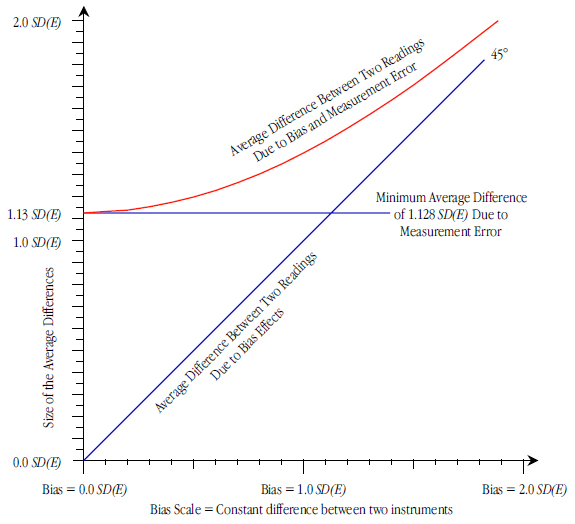Figure 2: The average difference between two readings

When we combine the effects of both bias and measurement error we find that the average difference between two readings of the same thing by two instruments will follow the curve in figure 2. The points that define the curve of figure 2 are tabled in figure 3.Figure 3: Bias and corresponding average differences in SD(E) units

Figure 2 shows that there is a region where measurement error is the dominant effect and that there is a region where bias effects dominate. The crossover point between these two regions occurs at the point where the two straight lines cross. Here the bias is equal to 1.128 SD(E) and from figure 3 the average difference between pairs of readings by the two instruments will be 1.467 SD(E).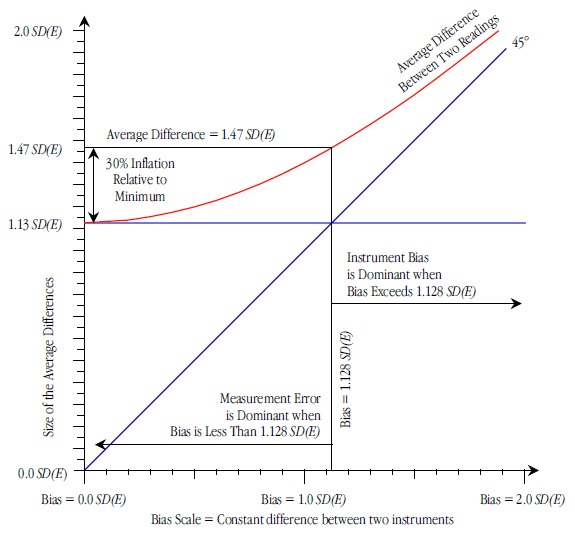Figure 4: The averages between two readings

On the left side of figure 4, where the instrumental bias is less than 1.128 SD(E), the average distance between readings of the same thing using the two instruments will always be within 30 percent of the minimum value.

On the right side of figure 4, where the instrument bias exceeds 1.128 SD(E), the bias will begin to create a systematic difference between the measurements from the two instruments. Here the instruments will no longer be equivalent in practice.

So, our criterion for practical equivalence becomes one of having an instrumental bias that is smaller than 1.128 SD(E).

The three instruments

In figure 1 we have 30 determinations of the value of a standard with instrument A. Figure 5 shows the consistency charts for 30 determinations of the same standard using instruments B and C.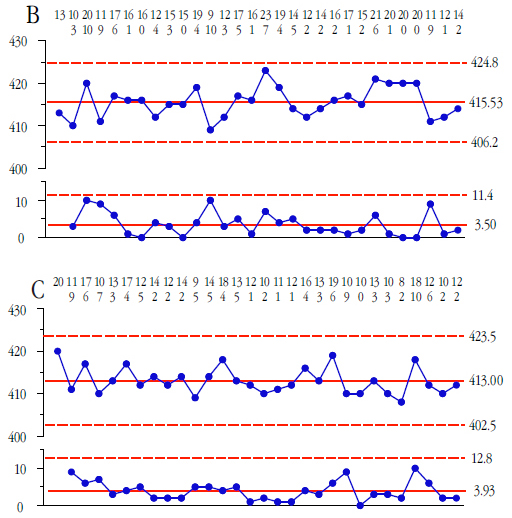Figure 5: Consistency charts for 30 repeated measurements of a single standard with Inst. B and Inst. C

The average range for instrument B is 3.50 units, thus the probable error is estimated to be 2.1 units. The average range for instrument C is 3.93 units, giving a probable error of 2.4 units. Earlier we found a probable error of 2.5 units for instrument A. The similarity of these probable errors suggests that these three instruments can be considered to have equivalent amounts of measurement error. A way to test this idea will be given in next month’s column.

For purposes of checking for detectable instrument bias we shall treat these three sets of data as k = 3 subgroups of size n = 30. (The demonstrated consistency of the instruments justifies this arrangement of these data.) Instrument A has an average of 415.57 and a global standard deviation statistic of 3.151. Instrument B has an average of 415.53 and a global standard deviation statistic of 3.598. Instrument C has an average of 413.00 and a global standard deviation statistic of 3.569. Each of these standard deviations has 29 degrees of freedom.

Thus, the pooled variance estimate of SD(E) is found by squaring each standard deviation statistic, averaging these values, and taking the square root. This pooled variance estimate has k(n–1) = 87 degrees of freedom and is:Thus, from above, we would expect duplicate readings made with the same instrument to differ by an average of 1.128 * 3.446 = 3.9 units.

Detecting bias effects

Our methodology for determining if two or more instruments are equivalent in practice shall be the analysis of means (ANOM). Here we have three subgroups of size 30, and want to compare the three averages. We shall use the traditional alpha level for one-time analyses of five percent. In this case, the formula for the detection limits for a 5-percent ANOM can be written as: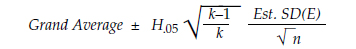The Grand Average is 414.70.

The ANOM scaling factor H.05 is found in the tables at the end of this paper. It depends upon the alpha level of 5 percent, the number of averages being compared, k = 3, and the degrees of freedom for the estimate of dispersion, 87 d.f. Rather than interpolating for 87 d.f., we round the 87 down to the table entry of 60 and use H.05 = 2.394.

Our estimate of the standard deviation of measurement error was 3.446 units, and the subgroup size is n = 30. Thus our 5-percent ANOM detection limits are: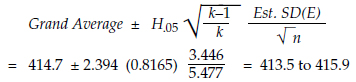Figure 6: ANOM for instrument bias

Here we find that there is a detectable bias effect between Instrument C and Instruments A and B. This gives us a license to estimate this bias. The average for Instruments A and B is 415.5 so readings from Instrument C average 2.5 units less than those from Instruments A and B. This bias is very likely to be real, but is it of practical importance?

Assessing the practical importance of the bias

As we found earlier, the average difference between two readings made with the same instrument is 1.128 SD(E) = 3.9 units. Our detected instrumental bias of 2.5 units is smaller than this minimum average difference. We can use the table in figure 3 to characterize the effect of this bias. The estimated bias of 2.5 units is only 73 percent as large as the estimate of SD(E) = 3.446.Interpolating in figure 3 we find that this bias corresponds to an average difference between a reading from instrument C and a reading from one of the other instruments of 1.274 SD(E)  =  4.4 units. Since these readings are recorded to whole numbers of units the instrumental bias will usually be lost in the round-off. (Two readings from the same instrument will differ by an average amount that rounds off to 4 units, while two readings from different instruments having a bias of 2.5 units will also differ by an average amount that rounds off to 4 units.

When your measurement systems fall on the left side of figure 4 measurement error will dominate any bias present, even though you may have sufficient data to detect and estimate instrumental biases. Just because a bias is detectable does not make it of practical importance.

Would recording the values to a tenth of a unit help? No. With an estimated standard deviation of 3.446 units the probable error for these instruments is estimated to be 2.33 units. The range of appropriately sized measurement increments will depend upon this probable error:Therefore recording values to a tenth of a unit would be an exercise in recording noise. These readings are recorded to a whole number of units, and they could even be rounded off to the nearest multiple of five units without causing any serious degradation in the quality of these readings.

But could we adjust the readings from instrument C? As outlined above, such an adjustment would have a minimal impact upon the quality of the readings. Adjusting the readings from instrument C would minimally reduce the average difference between two measurements of the same thing by different instruments by 13 percent (from 4.4 units to 3.9 units). So, if the adjustment is easy and economical, and if you are facing a tight tolerance, it is not incorrect to adjust the readings from instrument C after the fact by this known bias of 2.5 units. However, in most cases it is not necessary to do so.

Should we recalibrate instrument C? No. Since measurement error dominates the bias effect it is unlikely that a recalibration would actually result in a smaller bias. It is worth noting that all three consistency charts show that measurements of the same standard can vary by up to ± 10 units. Attempting to recalibrate when the bias is less than 1.128 SD(E) will be an exercise in frustration because measurement error is likely to result in the creation of new biases as you seek to remove the old biases.

Summary

An operational definition has to have three parts: a criterion to be used, a method for testing compliance to the criterion, and a way of interpreting the test results. The criterion for practical equivalence for instruments having similar amounts of measurement error is for biases to be less than 1.128 SD(E). (We will consider a way to check for having similar amounts of measurement error next month.) The methodology for detecting bias is the traditional ANOM. And the decision rule is contained within the criterion and technique. A detectable bias is a problem to be solved only when that bias substantially exceeds 1.128 SD(E).

The eighth axiom of data analysis is that you must detect a difference before you can legitimately estimate that difference, and only then can you assess the practical importance of that difference. All statistical procedures are designed to detect differences in spite of the noise present in the data. Given enough data we can detect differences that are too small to have any practical importance. The criterion offered here provides a way to assess the practical importance of instrumental biases.

Postscript

Those who are interested may verify the entries in figure 3 as follows. Generate a set of standard normal random observations and consider these to be repeated measurements of the same thing (so that the variation represents nothing but measurement error, making SD(E) = 1). Now pair these observations up and think of the first member of each pair as “observation A” and the second member as “observation B.” The average of the absolute values of the differences [A–B] of all of these pairs should be very close to 1.128. This corresponds to the no bias condition. Now add a fixed amount to each observation B (the bias amount) and recompute the absolute values of the differences between A and (B + bias) and average over all such pairs. The average absolute values for a given bias amount will correspond to the entries in figure 3.

Tables for ANOM

The following tables are excepted from more extensive tables given in Analyzing Experimental Data, by Donald J. Wheeler (SPC Press, 2013) and are used with permission. These values are appropriate for use with any unbiased within-subgroup estimate for the standard deviation of the k averages being compared. The general formula for computing the ANOM detection limits is: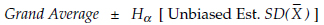Common unbiased estimators of the standard deviation of the subgroup averages are given in figure 7 for data arranged into k subgroups of size n.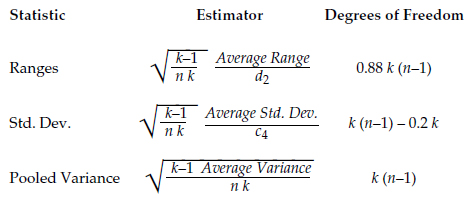Figure 7: Unbiased estimators for SD(Averages)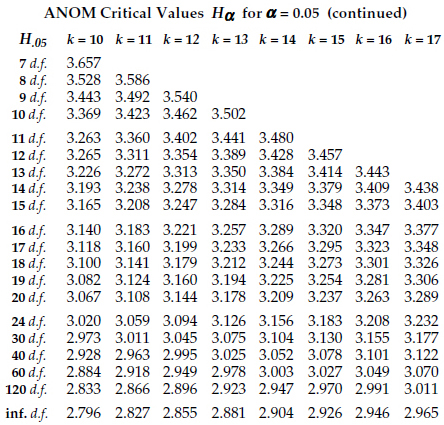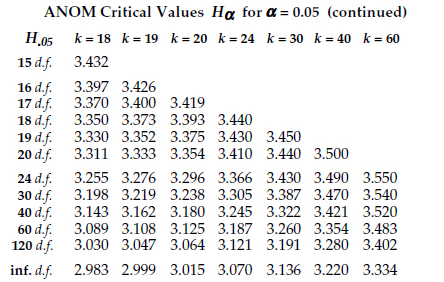DiscussDonald J. Wheeler

Dr. Donald J. Wheeler is a Fellow of both the American Statistical Association and the American Society for Quality, and is the recipient of the 2010 Deming Medal. As the author of 25 books and hundreds of articles, he is one of the leading authorities on statistical process control and applied data analysis. Find out more about Dr. Wheeler’s books at www.spcpress.com.

Dr. Wheeler welcomes your questions. You can contact him at djwheeler@spcpress.comJames Beagle III

James Beagle III is a lapsed CQE, CRE, and C6SBB (asking the wrong questions and obsessing about impractical concepts, before realizing what is important) with 35+years experience in process, quality, and reliability engineering. He primarily works with a large manufacturing base supplying data storage component products, where he develops tools for data analysis, designs experiments for product improvements, and works on product and process qualification. In his free time he works on numerical modeling (and is also known to have guitars at home). He can be reached at james@idealstates.net.